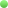Reply – Multiple VAO and VBO with JOGL
Subject
Message
or Cancel
Multiple VAO and VBO with JOGL
— byChen norris
Hello everyone,

This is my very first post in here about JOGL so sorry in advance if I missed a step before posting in here.
The problem I'm facing deals with VAO/VBO comprehension. As an example, I'd like to render a square in very specific (and not optimized) way : I would like to render two triangles with two different VAOs. The structure of my code looks like this :

INIT :
• creation of two VAOs (one for each triangle),
• creation of three VBOs (position, color and index). As far as I understood, the number of VBOs to create doesn't rely on the number of VAOs that's why I don't need to create six VBOs but only as many VBOs as I have attributes (a VBO for the position, a VBO for the color and a VBO for the index, shared between all VAOs),
• binding of the first VAO, then buffering the first triangle's attributes (3 positions, 3 colors and 3 indices),
• binding of the second VAO, then buffering the second triangle's attributes (3 positions, 3 colors and 3 indices).
RENDERING :
• binding of the first VAO, call to glDrawElement method, and unbinding the first VAO,
• binding of the second VAO, call to glDrawElement method, and unbinding the second VAO.
My code for the init part is then the following :
// creation of two VAOs
int[] tempVertexArrayObject = new int;
gl3.glGenVertexArrays(2, tempVertexArrayObject, 0);
vaoId1 = tempVertexArrayObject;
vaoId2 = tempVertexArrayObject;

// creation of three VBOs
IntBuffer idArray = GLBuffers.newDirectIntBuffer(3);
gl3.glGenBuffers(3, idArray);
positionBufferId = idArray.get(0);
colorBufferId = idArray.get(1);
indexBufferId = idArray.get(2);

// binding and buffering the first VAO
gl3.glBindVertexArray(vaoId1);

// buffering positions
gl3.glBindBuffer(GL.GL_ARRAY_BUFFER, positionBufferId);
gl3.glBufferData(GL.GL_ARRAY_BUFFER, positionBuffer.capacity() * Buffers.SIZEOF_FLOAT, positionBuffer, GL.GL_STATIC_DRAW);
gl3.glEnableVertexAttribArray(positionHandler);
gl3.glVertexAttribPointer(positionHandler, 3, GL.GL_FLOAT, false, 0, 0);
gl3.glBindBuffer(GL.GL_ARRAY_BUFFER, 0);

// buffering colors
gl3.glBindBuffer(GL.GL_ARRAY_BUFFER, colorBufferId);
gl3.glBufferData(GL.GL_ARRAY_BUFFER, colorBuffer.capacity() * Buffers.SIZEOF_FLOAT, colorBuffer, GL.GL_STATIC_DRAW);
gl3.glEnableVertexAttribArray(colorHandler);
gl3.glVertexAttribPointer(colorHandler, 4, GL.GL_FLOAT, false, 0, 0);
gl3.glBindBuffer(GL.GL_ARRAY_BUFFER, 0);

// buffering indices
gl3.glBindBuffer(GL.GL_ELEMENT_ARRAY_BUFFER, indexBufferId);
gl3.glBufferData(GL.GL_ELEMENT_ARRAY_BUFFER, indexBuffer.capacity() * Buffers.SIZEOF_INT, indexBuffer, GL.GL_STATIC_DRAW);

// binding and buffering the second VAO + buffering positions + ...
// same as before, but gl.glBindVertexArray(vaoId2) instead of gl.glBindVertexArray(vaoId1), and with other buffers
// that contain data for the other triangle
My code for the rendering part is the following :
// Drawing the first triangle
gl3.glBindVertexArray(vaoId1);
gl3.glDrawElements(GL3.GL_TRIANGLES, 3, GL3.GL_UNSIGNED_INT, 0);
gl3.glBindVertexArray(0);

// Drawing the second triangle
gl3.glBindVertexArray(vaoId2);
gl3.glDrawElements(GL3.GL_TRIANGLES, 3, GL3.GL_UNSIGNED_INT, 0);
gl3.glBindVertexArray(0);
Of course, I didn't put the part where I clear my background, I enable depth test, ...
My problem is that only the second triangle is drawn, as if buffering data when VAO #2 is binded buffers data for VAO #1. Another strange behavior I can see is that when I only call the Drawing the first triangle instructions, I can see my second triangle.

Any advice on something I misunderstood or on something I may do wrong ?